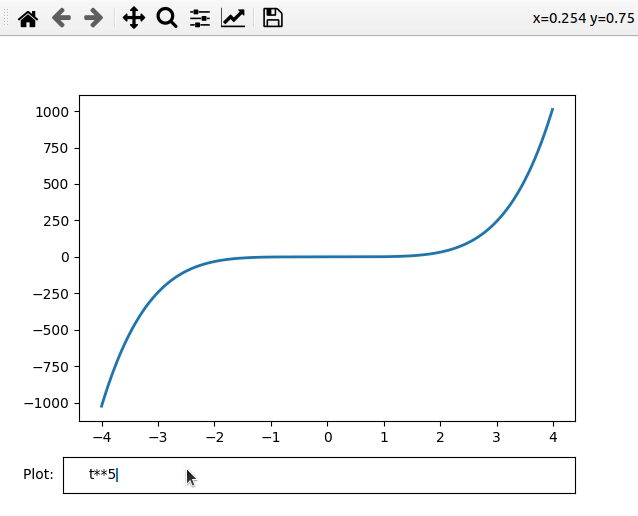# Matplotlib – Textbox Widgets

• Last Updated : 17 Feb, 2022

In this article, we are going to see matplotlib’s textbox widget. Matplotlib is a plotting library for the Python programming language. In this article, we will try to plot a graph for different powers(e.g. t^2, t^3, t^9, etc.) using textbox widget.

The Textbox is a widget that accepts input from the user. Input can also be formulas so that we can generate a graph based on those formulas. To use this widget we use TextBox() function.

It accepts two parameters

• Figure i.e. graph to use.
• Text to display with textbox i.e. Label to textBox.

Below is the implementation of this widget:

## Python

 `# Import modules``import` `numpy as np``import` `matplotlib.pyplot as plt``from` `matplotlib.widgets ``import` `TextBox` `# Adjust illustration``fig, ax ``=` `plt.subplots()``fig.subplots_adjust(bottom``=``0.2``)` `t ``=` `np.arange(``-``4.0``, ``4.0``, ``0.01``)``l, ``=` `ax.plot(t, np.zeros_like(t), lw``=``2``)` `# Function to plot graph``# according to expression``def` `visualizeGraph(expr):``    ``ydata ``=` `eval``(expr)``    ``l.set_ydata(ydata)``    ``ax.relim()``    ``ax.autoscale_view()``    ``plt.draw()` `# Adding TextBox to graph``graphBox ``=` `fig.add_axes([``0.1``, ``0.05``, ``0.8``, ``0.075``])``txtBox ``=` `TextBox(graphBox, ``"Plot: "``)``txtBox.on_submit(visualizeGraph)``txtBox.set_val(``"t**5"``)` `# Plot graph``plt.show()`

Output:Explanation:

In the above program, we created a graph for t2 as by default graph. In txtBox labelled as Plot: we can enter the formula we want to view the graph for.

My Personal Notes arrow_drop_up